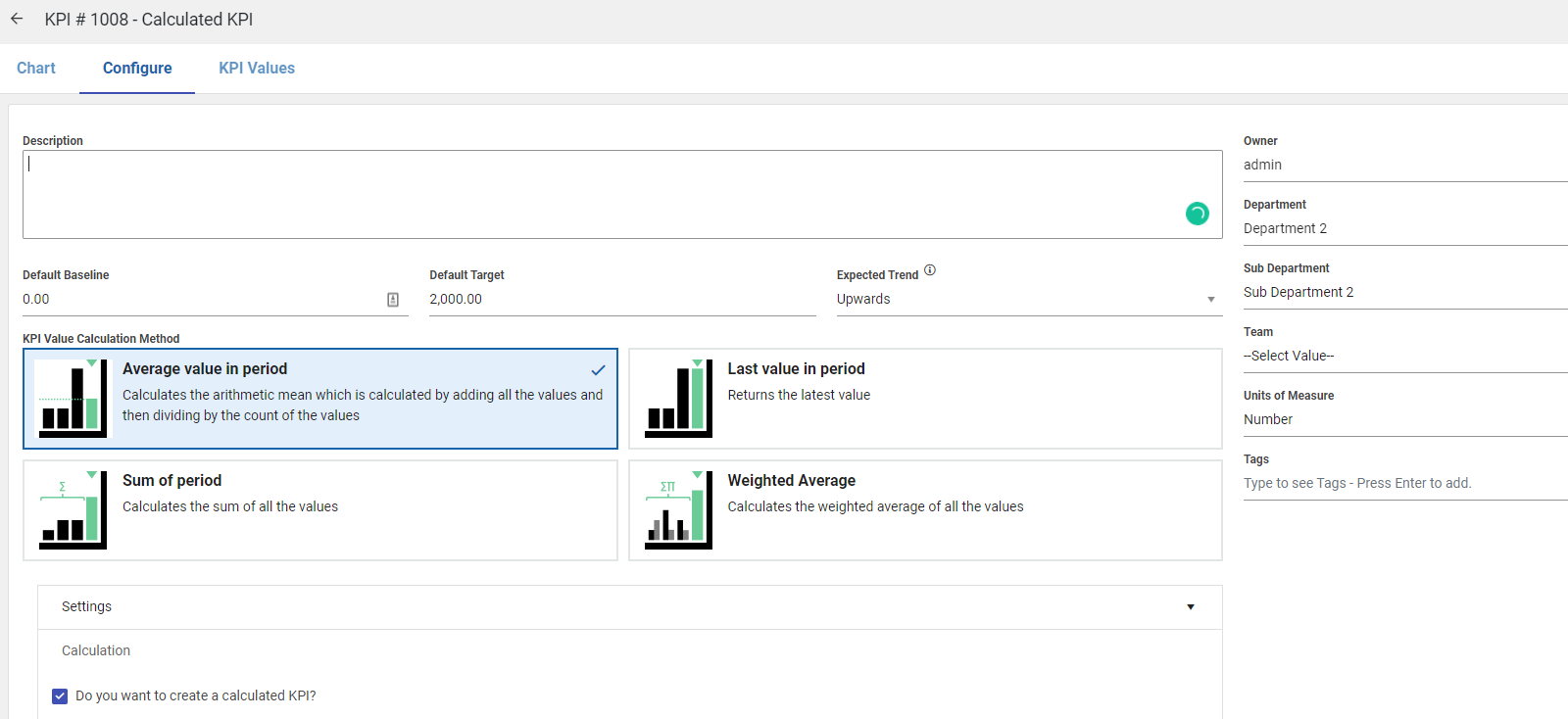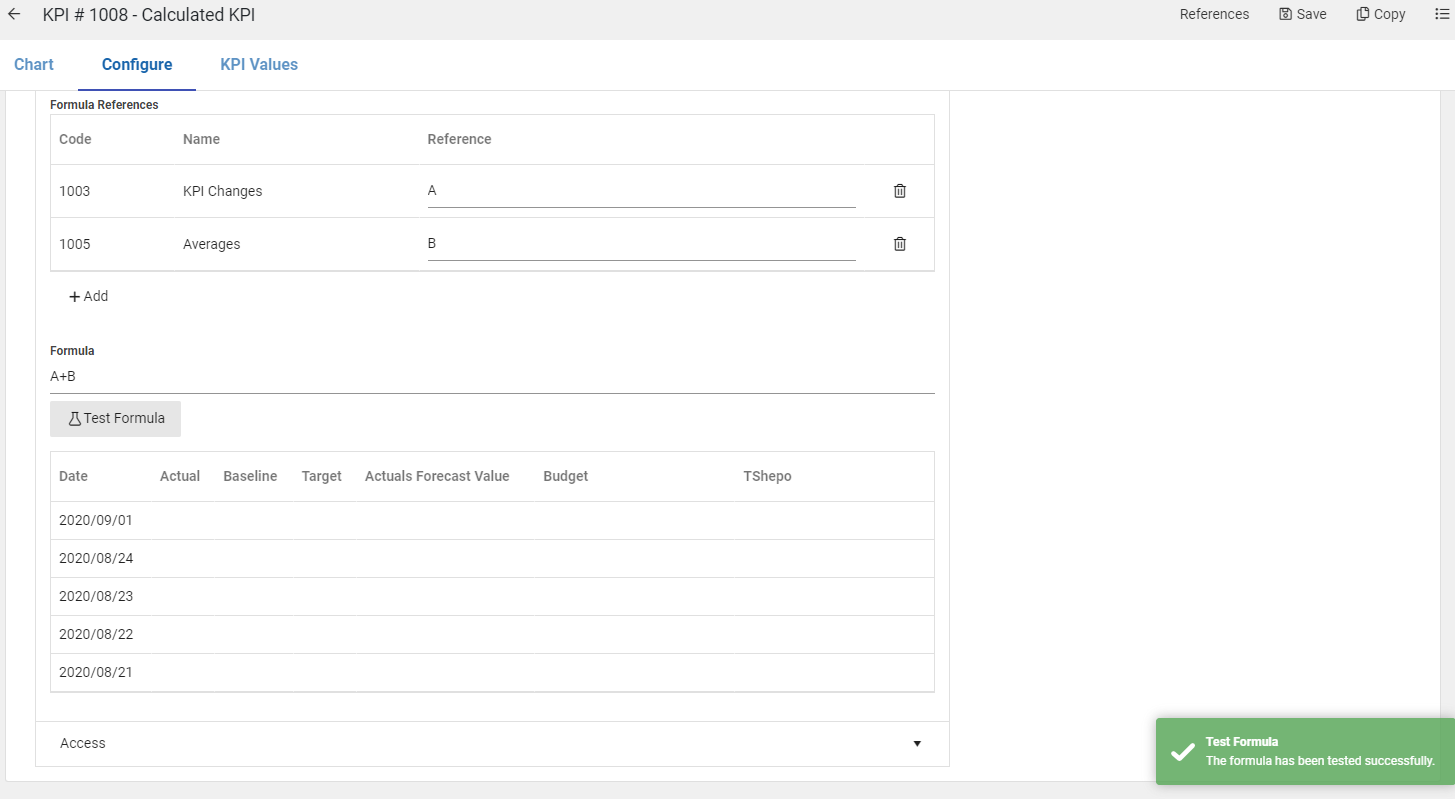Calculated KPIs are created the same way as normal KPIs, on the KPI List click on Add and mark the KPI as calculated as displayed below:

It is important to note the formula is applied on the Date match.• To mark the KPI as calculated you need to click on the checkbox highlighted Green above.
• You can then add the KPI(s) that will be used as input KPIs, by clicking the Add button under the calculation section. (Minimum requirement is that the input KPI should at least have one value)
• You will need to specify a formula that will be used to generate the values for the calculated KPI.

We recommend clicking on Test Formula for validation and to confirm if the produced values are as desired.Since the values on the Calculated KPI are driven by the input KPIs, every update done on an input KPI forces a recalculation of the Calculated KPI's values(Targets and Actuals)

Importantly, note the formula is applied on date match meaning. if you have a calculated KPI with the formula KPI A/KPI B depending on the selected Measurement Type i.e. The cumulative measurement types will calculate Weekly and Monthly values as the sum(KPI A/ KPI B) not sum(KPI A)/ sum(KPI B).